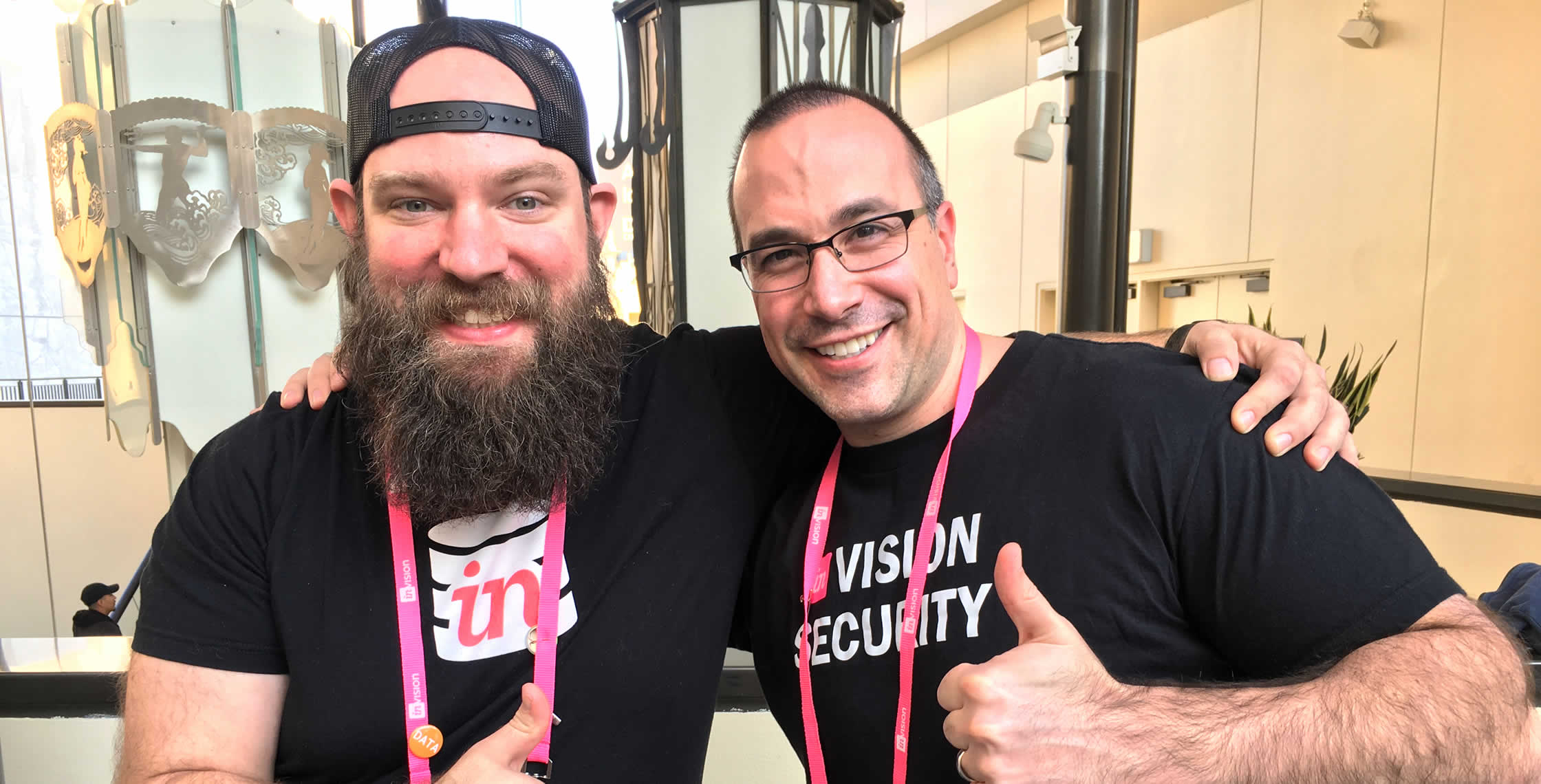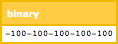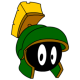Ben Nadel at InVision In Real Life (IRL) 2018 (Hollywood, CA) with: Jesse Dearing ( @JesseDearing )

# Creating Signed Java Byte Values Using ColdFusion Numbers

By on
Tags:

For the last two days, I've been baffled as to why I couldn't create a Byte from 8 bits. After much head-banging, I discovered that Java bytes are signed. This means that the 8th bit doesn't represent 128, but rather -128. In ColdFusion, bit-wise functions (such as bitAnd() and bitOr()) return long integers, not bytes. This makes creating signed Java bytes a bit problematic.

To demonstrate the issue, let's try to create a signed Java byte that represents the value, -100:

``````<cfscript>

// In Java, a byte is a signed value that can hold between -128 and 127 (inclusive).
// This means that -100 is -128 (first bit on) + 28. And since 28 is 00011100, it
// means that -100 is (10000000 | 00011100).
input = inputBaseN( "10011100", 2 );

// Now, if we try to cast this value to a byte, we get the error:
// ---
// coldfusion.runtime.Cast\$OutOfBoundsException: Cannot convert the value 156.0
// to byte because it cannot fit inside a byte
// ---
// The problem is that our input is a 32-bit Number, not a BYTE; so, it's not
// signed, which means that the most significant bit of the first octet is not
// the signed bit, but rather the 128 bit, which means that our input is really
// (128 + 28) = 156, which is outside the range of the signed Java byte.
byte = javaCast( "byte", input );

</cfscript>
``````

Here, we're using the inputBaseN() function to describe the number using a bit-string that should represent -100. However, when we try to cast that value to a native Java Byte, we get the following ColdFusion error:

coldfusion.runtime.Cast\$OutOfBoundsException: Cannot convert the value 156.0 to byte because it cannot fit inside a byte

The problem is that inputBaseN() - in addition to all the bit-wise functions - returns a Numeric value, not a Byte. This means that the 8th bit doesn't represent the sign, but rather the value, 128.

Luckily, just the other day, Darren Whorton was telling me how bits work; and, more specifically, how signed values work and, what two-compliment is. Using what he told me, I was able to figure out that I could represent the same negative value (ie, -100) in Integer format by extending the sign-bit from the 8th bit all the way through to the 32nd bit.

By padding with 1s, it means that the negative value we want is properly represented using 4 octets instead of just one. And, once we have that negative number, we can cast it to a native Java Byte:

``````<cfscript>

// In Java, a byte is a signed value that can hold between -128 and 127 (inclusive).
// This means that -100 is -128 (first bit on) + 28. And since 28 is 00011100, it
// means that -100 is (10000000 | 00011100).
input = inputBaseN( "10011100", 2 );

// However, the inputBaseN() function returns a 32-bit Number, not a byte. Which
// means that it doesn't know that the 8th bit is the sign bit for a byte. As such,
// if we want to cast to a byte, we need to make the *Number* negative, not just the
// first octet. Using two's compliment, we can do this by turning on the other 3 octets.
negativeMask = inputBaseN( ( "11111111" & "11111111" & "11111111" & "00000000" ), 2 );

// Check to see if 8th bit is turned on (we know it is, but putting this here to
// demonstrate that it's actually true).
if ( bitMaskRead( input, 7, 1 ) ) {

// To get our "byte" input to be reprsented as a Number, we have to OR it with
// the negative mask. This will get the negative property of the 8th bit extended
// through the rest of the 4-octet value.
negation = bitOr( negativeMask, input );

// Now, we can cast this to a signed-byte that represents -100.
byte = javaCast( "byte", negation );

// And, to demonstrate this more clearly, I'm going to cast to a byte-array
// (ie, a binary value) where we can see the -100 being output.
writeDump( javaCast( "byte[]", [ byte, byte, byte, byte, byte ] ) );

}

</cfscript>
``````

In the above code, once I have the native byte, I'm then creating a Byte Array (ie, a binary value), so that the output is more colorful, yay!:As you can see, the rendered value is a byte-array composing a collection of signed-bytes that represent -100 (each).

This was quite a stumper! Thank goodness Darren Whorton came along, or I would have never figured this out. Now, I can go back and fix my Base32 encoding experiment, which was breaking for ASCII values over 127.

Want to use code from this post? Check out the license.Hey Ben, thanks for the shout-out! Thrilled I could help.

It's always fascinating to see how Coldfusion and Java play together, as it's often undocumented. Coldfusion is very loosely typed, which has its upsides and downsides (more upsides in my opinion). But in this case, it's important to know what type you're working with... specifically that the inputBaseN() function returns a 32-bit signed integer.

Made me wonder what would happen if you tried to give inputBaseN() something larger than 32 bits.

inputBaseN("00000001" & "FFFFFFFF",16)

The answer is, it truncates to 32 bits and returns -1, which is what the lower half of the argument represents taken by itself (the full 64-bit value in decimal is 8589934591). No out of bounds exception is thrown.

Now, Coldfusion supports larger integers. <cfset x = 8589934591> works fine. But then if you try to call FormatBaseN(x,16), you get an out of bounds exception. So, looks like all the "baseN" functions are 32-bit only. Interesting!@Darren,

Yeah, the loosely typed stuff means that I generally don't have to think about it... until it starts breaking :D The docs are also a little confusing. For the bit-functions, it states:

> The bitwise AND of two long integers.

What is a "long integer"? I assume what they mean is that even though your dealing with "bits", the return value is a full 32-bit integer, not an 8-bit integer.

This stuff is starting to affect my ability to sleep! I keep thinking of bits and shifting. But, it's definitely shining some light on a part of computer science that I almost never think about.

What's really interesting is that a byte-array with two bytes is sometimes rendered as a two-character string (if both bytes are <= 127) OR, a one-character string, if the bytes are negative (>127). I guess is the magic that is UTF-8 encoding and how it figures out how many bytes are required to make a "character." Really fascinating.A long integer in Java is actually 64-bits. Java has byte (8-bit), short (16-bit), int (32-bit) and long (64-bit) integers, and then BigInteger is arbitrary length, theoretically only limited by the memory of the machine you're running on (which is kinda crazy).

Oh man, don't get me started on UTF-8... :) Anywhere from 1 to 4 bytes to encode a single character. Basically you read a byte, and if it starts with 0 it's a one-byte character. If it starts with 10, it's a two-byte character, so read two bytes, and then extract specific bits from those bytes to get the character code. If it starts with 110, it's a three-byte character, etc. It's really quite elegant and super efficient, but it can get frustrating if you need to do byte-level operations. The Wikipedia page has a good summary: http://en.wikipedia.org/wiki/UTF-8#Description

But, we digress. :)@Darren,

I'm just glad that ColdFusion has charsetEncode() and charsetDecode() to convert binary->string and string->binary, respectively. That's the only way I could possibly do this stuff :)